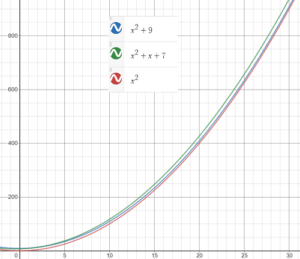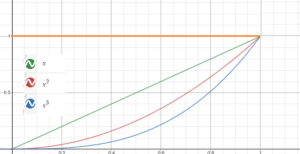# Big O Notation (Landau’s Symbol)/Order of a Function

The symbol O , called Big O or Big Oh is used to describe the asymptotic behavior of a function as x grows to infinity (i.e. the asymptotic upper bound). It gives you an idea of how fast or slow a function grows (or decays) and is defined in terms of limits, both in calculus and in other areas like computer science (Langer, 2020).

The “O” indicates the order of a function: For example, the notation O(1) means “of order at least 1” or “is of the same order as 1”. The order refers to the growth rate of a function (not to be confused with the order of continuity).

The notation was invented by German mathematician Edmund Landau, so is sometimes called Landau’s symbol, although that particular term usually refers to both Big O and little o.

## A Graphical Explanation

The notation f(x) = O(g(x)) indicates the function is bounded by a multiple of g(x) as it tends to large inputs; This is usually as the function tends to infinity, but it can also be as it tends to zero. In other words, after (or before) a certain x-value, the function f(x) is always smaller than constant multiples of g(x). In terms of inequalities, f ∈ O(g(x)) means that, given at least one constant k > 0, you can find another constant a so that 0 ≥ f(x) ≥ k g(x) holds for all x > a.

A function’s growth is determined by the term with the highest order. For example, in the following set of functions, the highest order term is x2:

• f(x) = x2 + 9
• f(x) = x2 + x + 7
• f(x) = x2

All of these functions grow as fast as each other as you head to infinity, because values of x2 are much larger than values of x or x + 1. So we can say, for example, that f(x) = x2 + x + 7 is O(x2), as the following image shows:What about small values of x? We might be interested in how a function behaves as it tends to zero instead of infinity. The following graph shows how a set of functions behaves as they tend to x = 0 (graphed with Desmos graphing calculator):For small values of x, the graph shows that 1 >> x >> x2 >> x3. So we can say, in big O notation, that f(x) = O(1) as x → 0.

## Where is Big O Notation Used?

Big O notation is widely used in many areas of mathematics (including calculus), complexity theory, and computer science. In general, it simply shows the asymptotic behavior of a function. But this simple tool has a myriad of uses. For example, it can:

• Establish an upper bound for the growth of a function; In this case, big Omega (Ω) establishes a lower bound (Scheinerman, 2012).
• In computer science, classify algorithms according to how fast (or slow) they run or how much space they require to run as the inputs grow (Mohr, 2014). In other words, it describes the upper bound for an algorithm’s complexity.
• Describe the vanishing of an approximation error,
• To conveniently rewrite Taylor’s formula (Callahan, 2010)

Depending on where you’re using big O notation, it either means order of vanishing or order of magnitude.

## References

Callahan, J. (2010). Advanced Calculus: A Geometric View. Springer, New York.
Langer, M. (2020). An analogy from Calculus: limits. Retrieved October 13, 2020 from: http://www.cim.mcgill.ca/~langer/250/bigO_Omega_Theta.pdf
Mohr, Austin. “Quantum Computing in Complexity Theory and Theory of Computation” (PDF). p. 2. Retrieved 7 June 2014.
Scheinerman, E. (2012). Mathematics: A Discrete Introduction. Cengage Learning,

CITE THIS AS:
Stephanie Glen. "Big O Notation (Landau’s Symbol)/Order of a Function" From StatisticsHowTo.com: Elementary Statistics for the rest of us! https://www.statisticshowto.com/big-o-notation-landaus-symbol/